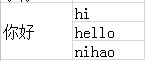## POI这种读取合并单元格如何实现！！！！！！！！！！！！！0

0

```        /**
* 获取合并单元格的值
* @param sheet
* @param row
* @param column
* @return
*/
public String getMergedRegionValue(Sheet sheet ,int row , int column){
int sheetMergeCount = sheet.getNumMergedRegions();

for(int i = 0 ; i < sheetMergeCount ; i++){
CellRangeAddress ca = sheet.getMergedRegion(i);
int firstColumn = ca.getFirstColumn();
int lastColumn = ca.getLastColumn();
int firstRow = ca.getFirstRow();
int lastRow = ca.getLastRow();

if(row >= firstRow && row <= lastRow){

if(column >= firstColumn && column <= lastColumn){
Row fRow = sheet.getRow(firstRow);
Cell fCell = fRow.getCell(firstColumn);

return getCellValue(fCell) ;
}
}
}

return null ;
}

/**
* 判断指定的单元格是否是合并单元格
* @param sheet
* @param row
* @param column
* @return
*/
public boolean isMergedRegion(Sheet sheet , int row , int column){
int sheetMergeCount = sheet.getNumMergedRegions();

for(int i = 0 ; i < sheetMergeCount ; i++ ){
CellRangeAddress ca = sheet.getMergedRegion(i);
int firstColumn = ca.getFirstColumn();
int lastColumn = ca.getLastColumn();
int firstRow = ca.getFirstRow();
int lastRow = ca.getLastRow();

if(row >= firstRow && row <= lastRow){
if(column >= firstColumn && column <= lastColumn){

return true ;
}
}
}

return false ;
}

/**
* 获取单元格的值
* @param cell
* @return
*/
public String getCellValue(Cell cell){

if(cell == null) return "";

if(cell.getCellType() == Cell.CELL_TYPE_STRING){

return cell.getStringCellValue();

}else if(cell.getCellType() == Cell.CELL_TYPE_BOOLEAN){

return String.valueOf(cell.getBooleanCellValue());

}else if(cell.getCellType() == Cell.CELL_TYPE_FORMULA){

return cell.getCellFormula() ;

}else if(cell.getCellType() == Cell.CELL_TYPE_NUMERIC){

return String.valueOf(cell.getNumericCellValue());

}

return "";
}```